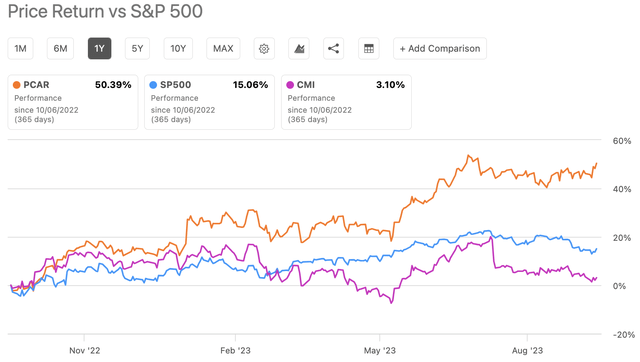## Thursday, December 15, 2022

### J.M. Smucker's Low Correlation With The Vanguard S&P 500 Index ETF

J.M. Smucker is known for its iconic and timeless consumer staples brands (Exhibit 1)

Note: Click on each image in this blog post to view an enlarged version

Exhibit 1:Brands Owned by J.M. Smucker & Co. (Source: J.M. Smucker)

Here's the histogram of monthly returns for J.M. Smucker between June 2019 and November 2022 (Exhibit 2).

Exhibit 2: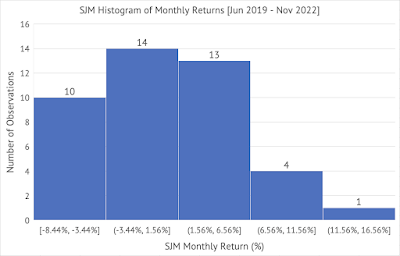J.M. Smucker (SJM) Histogram of Monthly Returns (Source: Data provided by IEX Cloud, author calculations & graph using Microsoft Excel)

The average monthly return for J.M. Smucker is less than the Vanguard S&P 500 Index ETF (Exhibit 3).
Exhibit 3: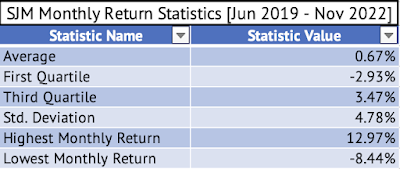J.M. Smucker Monthly Return Statistics - Average, First Quartile, Third Quartile, Standard Deviation, Highest Monthly Return, Lowest Monthly Return (Source: Data provided by IEX Cloud, author calculations & graph using Microsoft Excel)

J.M. Smucker had a lower standard deviation than the Vanguard ETF during this period (Exhibit 4). A company with a lower standard deviation than the well-diversified ETF, a measure of volatility, is an infrequent occurrence.

Exhibit 4: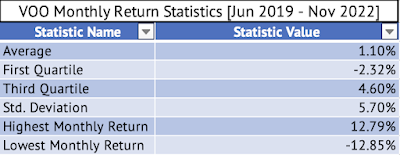Vanguard S&P 500 Index ETF Monthly Return Statistics - Average, First Quartile, Third Quartile, Standard Deviation, Highest Monthly Return, Lowest Monthly Return (Source: Data provided by IEX Cloud, author calculations & graph using Microsoft Excel)

Here's a graph of the monthly returns of the Vanguard ETF (x-axis) and J.M.Smucker (y-axis) with the fitted regression line (Exhibit 5).
Exhibit 5: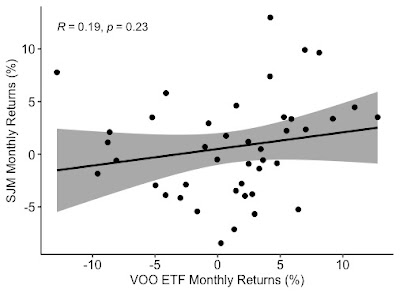Monthly Return Graph of the Vanguard S&P 500 Index ETF and J.M. Smucker (Source: Data provided by IEX Cloud, author calculations & graph using Microsoft Excel & RStudio)

The correlation of the monthly returns between June 2019 and November 2022 between the Vanguard ETF and J.M. Smucker is a low 0.19 (Exhibit 5). The fitted linear regression line has a p-value of 0.23, indicating that the relationship is insignificant at the 95% confidence interval.

The fitted linear regression line has a p-value of 0.23, indicating that the relationship is insignificant at the 95% confidence interval. Here's the output from the linear regression:

> summary(lmVOOSJM)

Call:
lm(formula = SJM_Monthly_Return ~ VOO_Monthly_Return, data = VOOandSJM)

Residuals:
Min        1Q    Median        3Q       Max
-0.089842 -0.034389  0.002403  0.022171  0.118077

Coefficients:
Estimate  Std. Error t value  Pr(>|t|)
(Intercept)        0.005011   0.007476   0.670    0.507
VOO_Monthly_Return 0.157911   0.130216   1.213    0.232

Residual standard error: 0.04755 on 40 degrees of freedom
Multiple R-squared:  0.03546, Adjusted R-squared:  0.01135
F-statistic: 1.471 on 1 and 40 DF,  p-value: 0.2324

The beta for J.M. Smucker is 0.15, but the high p-value is a concern. This beta (the coefficient of VOO_Monthly_Return) may not be the true value. Yahoo Finance has calculated a beta of 0.24.